# Sullivan conjecture

Jump to: navigation, search

A conjecture in homotopy theory usually referring to a theorem about the contractibility, or homotopy equivalence, of certain types of mapping spaces. These results are vast generalizations of two different but related conjectures made by D. Sullivan in 1972.

H.T. Miller [a1] achieved the first major breakthrough and is given credit for solving the Sullivan conjecture. This was published in 1984 and one version reads: The space of pointed mappings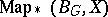from the classifying space of a finite groupto a finite CW-complexis weakly contractible. The mapping space has the compact-open topology.

An equivalent statement is that the space of unpointed mappings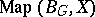is weakly homotopy equivalent to(under the same hypotheses onand).

These theorems are still true when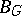is replaced by a CW-complex which has only finitely many non-zero homotopy groups, each of which is locally finite and wherecan be any finite dimensional CW-complex. This improvement is due to A. Zabrodsky.

Equivariant versions of the Sullivan conjecture come about by considering the question: How close does the natural mapping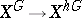come to being a homotopy equivalence? Here,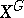is the fixed-point set of a group actionon the spaceand the homotopy fixed-point set is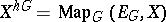, the space of equivariant mappings from the contractible space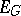on whichacts freely to the-space. Foracting trivially on, Miller's version of the Sullivan conjecture gives a positive answer to this question.

Another version of this question is that the fixed-point set of a-space localized at a prime numberis weakly homotopy equivalent to the homotopy fixed-point set ofacting on the-localization of. One proof of this result has been given by G. Carlsson, via the Segal conjecture [a2]. Miller also independently proved this result, and J. Lannes has a subsequent proof using his-functor (cf. also Lannes-functor).

These theorems have found many beautiful applications at the hands of the above-mentioned mathematicians, as well as W.G. Dwyer, C. McGibbon, J.A. Neisendorfer and C. Wilkerson, and S. Jackowsky, to name only a few.